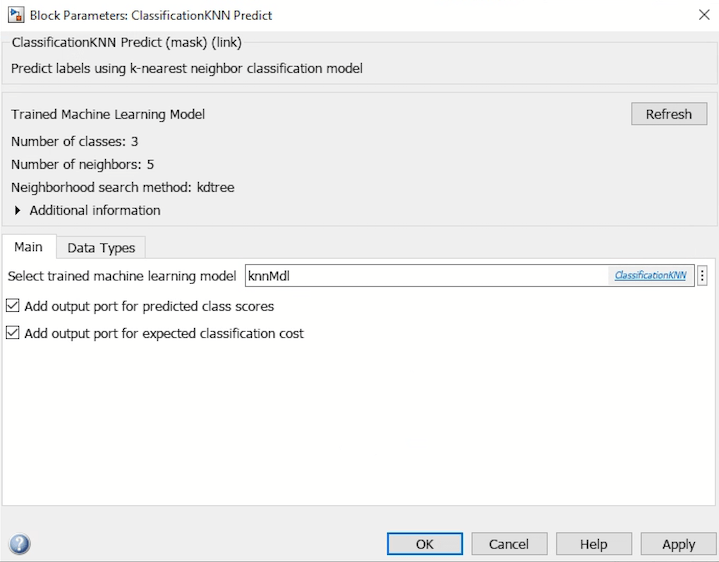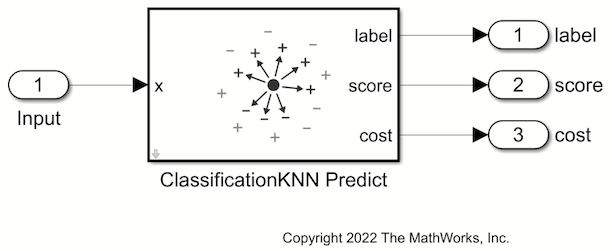# Predict Class Labels Using ClassificationKNN Predict Block

This example shows how to use the ClassificationKNN Predict block for label prediction in Simulink®. The block accepts an observation (predictor data) and returns the predicted class label, class score for the observation, and expected classification cost using the trained nearest-neighbor classification model.

### Train Nearest-Neighbor Classifier

Train a nearest-neighbor classifier, and assess the performance of the classifier on a test set.

Load the f`isheriris` data set. Create `X` as a numeric matrix that contains four petal measurements for 150 irises. Create `Y` as a cell array of character vectors that contains the corresponding iris species.

```load fisheriris X = meas; Y = species;```

For reproducibility of the partition, set the seed for the random number generator. Randomly partition observations into a training set and a test set with stratification, using the class information in Y. Use approximately 80% of the observations to train a nearest-neighbor model, and 20% of the observations to test the performance of the trained model on new data.

```rng("default") cv = cvpartition(Y,"HoldOut",0.2);```

Extract the training and test indices. Specify the training and test data sets.

```trainingInds = training(cv); testInds = test(cv); Xtrain = X(trainingInds,:); Ytrain = Y(trainingInds); Xtest = X(testInds,:); Ytest = Y(testInds);```

Train a 5-nearest neighbor classifier by using the `fitcknn` function. Standardize the noncategorical predictor data.

```knnMdl = fitcknn(Xtrain,Ytrain, ... NumNeighbors=5,Standardize=1)```
```knnMdl = ClassificationKNN ResponseName: 'Y' CategoricalPredictors: [] ClassNames: {'setosa' 'versicolor' 'virginica'} ScoreTransform: 'none' NumObservations: 120 Distance: 'euclidean' NumNeighbors: 5 Properties, Methods ```

`knnMdl` is a trained `ClassificationKNN` model.

Evaluate the performance of the classifier on the test set by computing the test set classification accuracy.

```testError = loss(knnMdl,Xtest,Ytest,LossFun="classiferror"); testAccuracy = 1 - testError```
```testAccuracy = 0.9333 ```

Create a new model using the ClassificationKNN Predict block. To create a new Simulink model, open the Blank Model template and add the ClassificationKNN Predict block from the Statistics and Machine Learning Toolbox™ library.

Double-click the ClassificationKNN Predict block to open the Block Parameters dialog box. Import a trained `ClassificationKNN` model into the block by specifying the name of a workspace variable that contains the model. The default variable name is `knnMdl`, which is the model you trained at the command line.

Click the Refresh button to refresh the settings of the trained model in the dialog box. The Trained Machine Learning Model section of the dialog box displays the options used to train the model `knnMdl`. Select the check boxes Add output port for predicted class scores and Add output port for expected classification cost to add the second (score) and third (cost) output ports in the block.Add one Inport and three Outport blocks, and connect them to the input and outputs of the ClassificationKNN Predict block.

The ClassificationKNN Predict block expects an observation containing four predictor values, because the model was trained using a data set with four predictor variables. Double-click the Inport block, and set Port dimensions to 4 on the Signal Attributes tab. To specify that the output signals have the same length as the input signal, set Sample Time to 1 on the Execution tab.

Create an input signal in the form of a structure array for the Simulink model. The structure array must contain these fields:

• `time` — The points in time at which the observations enter the model. The orientation must correspond to the observations in the predictor data. In this example, `time` must be a column vector.

• `signals` — A 1-by-1 structure array describing the input data and containing the fields `values` and `dimensions`, where `values` is a matrix of predictor data, and `dimensions` is the number of predictor variables.

```modelInput.time = (0:length(Ytest)-1)'; modelInput.signals(1).values = Xtest; modelInput.signals(1).dimensions = size(Xtest,2);```

Import the signal data from the workspace:

1. Open the Configuration Parameters dialog box. On the Modeling tab, click Model Settings.

2. On the left of the Configuration Parameters dialog box, click Data Import/Export. Then select the Input check box and enter `modelInput` in the adjacent text box.

3. On the left, click Solver. Under Simulation time, set Stop time to `modelInput.time(end)`. Under Solver selection, set Type to `Fixed-step`, and set Solver to `discrete (no continuous states)`.Click the Outport 1 block and set the block name to `label`. Similarly, change the Outport 2 and Outport 3 block names to `score` and `cost`, respectively.

### Open Provided Model

Instead of creating a new model, you can open the provided Simulink model `slexClassificationKNNPredictExample.slx`, which includes the ClassificationKNN Predict block. To access this model, you must open the example as a live script.

Open the Simulink model `slexClassificationKNNPredictExample.slx`.

```SimMdlName = "slexClassificationKNNPredictExample"; open_system(SimMdlName)```The `PreLoadFcn` callback function of `slexClassificationKNNPredictExample` includes code to load the sample data, train the nearest-neighbor model, and create an input signal for the Simulink model. If you open the Simulink model, the software runs the code in `PreLoadFcn` before loading the Simulink model. To view the callback function, in the Setup section on the Modeling tab, click Model Settings and select Model Properties. Then, on the Callbacks tab, select the `PreLoadFcn` callback function in the Model callbacks pane.

Simulate the Simulink model, and export the simulation outputs to the workspace. When the Inport block detects an observation, it places the observation into the ClassificationKNN Predict block. You can use the Simulation Data Inspector (Simulink) to view the logged data of an Outport block.

`simOut = sim(SimMdlName)`
```simOut = Simulink.SimulationOutput: tout: [30x1 double] yout: [1x1 Simulink.SimulationData.Dataset] SimulationMetadata: [1x1 Simulink.SimulationMetadata] ErrorMessage: [0x0 char] ```

Determine the simulated classification labels.

```outputs = simOut.yout; sim_label = outputs.get("label").Values.Data;```

Create a confusion matrix chart from the true labels (`Ytest`) and the labels predicted by the Simulink model (`sim_label`).

`confusionchart(string(Ytest),string(sim_label))`Large values on the diagonal indicate accurate predictions for the corresponding class.